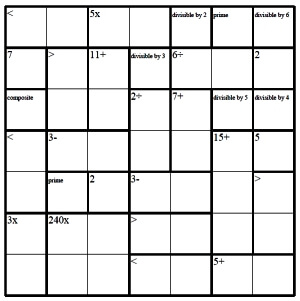Worksheets and No Prep Teaching Resources
Critical Thinking Puzzles
Make Puzzles

# edHelperKu KenKen Puzzles Puzzle Workbooks for Kids - Updated Each Month!

Like Sudoku puzzles, but with math, edHelperKu puzzles (also known as KenKen puzzles) can be customized with addition, subtraction, multiplication, division, and various combinations thereof. Unlike regular Sudoku puzzles, students use math clues provided in the puzzle itself to figure out which numbers should be entered into which boxes. As always, there are many customization options, including the math operations used with the puzzle, the puzzle's difficulty level, and its size. Once your students try edHelperKu puzzles, ordinary Sudoku will never satisfy again!edHelperKu Custom Puzzles

Supermix edHelperKu Puzzles
Supermix edHelperKu puzzles have it all - addition, multiplication, division, subtraction, greater than, less than, prime and composite numbers
Make custom edHelperKu supermix puzzles

Easier edHelperKu Supermixes
easiest supermix - 4 by 4 edHelperKu with 3 boxes filled-in (max cage size of 3)
4 by 4 edHelperKu with 1 box filled-in (max cage size of 3)
5 by 5 edHelperKu with 3 boxes filled-in (max cage size of 3)
6 by 6 edHelperKu with 3 boxes filled-in (max cage size of 3)

edHelperKu Supermix
4 by 4 edHelperKu supermix
5 by 5 edHelperKu supermix
6 by 6 edHelperKu supermix
7 by 7 edHelperKu supermix

Most Difficult edHelperKu Supermixes
4 by 4 edHelperKu supermix
5 by 5 edHelperKu supermix
6 by 6 edHelperKu supermix
7 by 7 edHelperKu supermix

Addition edHelperKu Puzzles
Make Custom edHelperKu addition puzzles
edHelperKu addition puzzles
edHelperKu addition puzzles with greater and less than
edHelperKu addition puzzles with greater and less than, divisible by, prime/composite

Subtraction edHelperKu Puzzles
Make Custom edHelperKu subtraction puzzles
edHelperKu subtraction puzzles

Addition and Subtraction Mixed edHelperKu Puzzles
Make Custom edHelperKu subtraction puzzles
edHelperKu addition and subtraction puzzles

Multiplication edHelperKu Puzzles
Make Custom edHelperKu multiplication puzzles
edHelperKu multiplication puzzles

Division edHelperKu Puzzles
Make Custom edHelperKu division puzzles
edHelperKu division puzzles
edHelperKu division puzzles with divisible by

Multiplication and Division edHelperKu Puzzles
Make Custom edHelperKu multiplication and division puzzles
edHelperKu multiplication and division puzzles

Daily Critical Thinking Skills - Get Mixed Puzzles at Once!

Have a suggestion or would like to leave feedback?
Leave your suggestions or comments about edHelper!